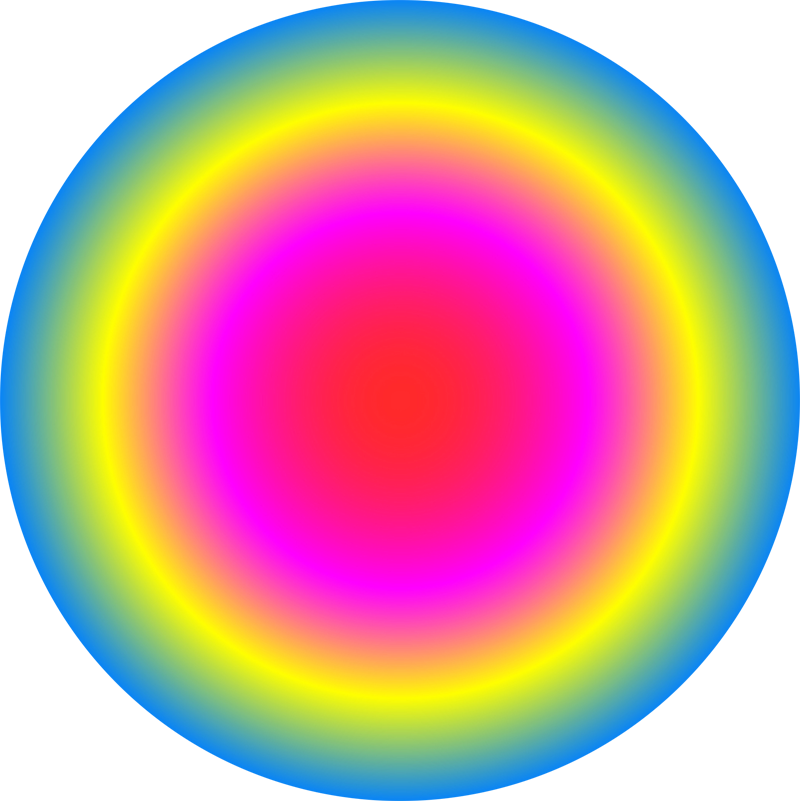Conor Lawless

Scientific Computing

data science, dynamic simulation modelling, genomics, interactive visualisation, dashboards, image & video analysis
e: cnr.lwlss@gmail.com
t: @cnrlwlss

Visualising correlation in an AR(1) process

Autoregression models are a type of stochastic, dynamic process. They are a mathematical representation of some value that varies with time, where the variation includes a random, unpredictable component. Using a computer to generate (pseudo-)random numbers, we can generate a set of simulated values across time that are consistent with this kind of model. Stochastic simulations aim to capture the random component in the process and so are usually different every time they’re run. ... Read more

Quantifying the strength & significance of genetic interactions

Recently, I have been analysing some functional genomics and drug screen datasets for clients. The techniques I use are similar to those I developed while working on and using a method called Quantitative Fitness Analysis (QFA) (Addinall et al. (2011), Lawless et al. (2010)). QFA is a method for screening the health of up to tens of thousands of microbial cell populations (examples in picture above). It is typically used to search for genes interacting with a gene of particular interest. ... Read more

Exactly when do days begin and end?

If you live anywhere away from the equator, you’ll be familiar with the fact that days are short in winter and long in the summer. There’s even a slight effect at the equator, but the farther away from the equator (e.g. the farther north you live in Europe) the more extreme this effect is. At the poles, there is a period in the summertime when the sun never sets, and similarly in winter, there is a period when the sun never rises. ... Read more

Translating maths into code: writing the tattoo function in Python

In a previous post I discussed a mathematical function which represents a range of different list-comprehensions and how their output can be visualised. Here I’ll describe in detail how we can evaluate this function on a computer, using the Python programming language as an example. Here is the mathematical notation for the tattoo function $t$ again: $$t(\theta, p) = \left\{ \sum_{k=-1}^{n}e^{\frac{2 \pi i k^p}{\theta}} \mid n \in -1,\dots,\theta \right\}$$ What should we expect from mathematical expressions in code? ... Read more

Mathematical art: tattoos & list-comprehensions

In 2007, as part of a series of collaborations with the visual artist James Johnson-Perkins, I made a video demonstrating the diversity of output possible from a fairly simple mathematical function (see function $t$ below). The video was displayed on large, external, urban screens in Derby, Nottingham and Leicester as part of the ‘Do billboards dream of electric sheep?’ exhibition. The shapes displayed in each of the frames of the video were generated by passing the frame number to a function like $t$: ... Read more